# npm

Have ideas to improve npm?Join in the discussion! »

## niims

1.0.3 • Public • Published

# niims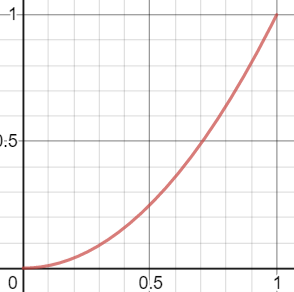## USING niims

``````const niims = require("niims");
``````

### HOW IT WORKS

This package nonlinearly (or linearly if you so choose) interpolates between two numbers. No matter the method of interpolation, three arguments are needed.

`````` linearProgress // A number between min and max that represents where to interpolate the output number. For example, assuming min and max are 0 and 1, 0.5 would transform into 0.25 when using parabolic interpolation, and 0.75 would transform into 0.56.
 min // The lowest number that can be interpolated between.
 max // The largest number that can be interpolated between.
``````

Here are some examples:

``````niims.load("parabolic")
niims.parabolic(0.5, 0, 1) // 0.25
niims.parabolic(50, 0, 100) // 25
niims.parabolic(0.5, 0, 1, {power: 3}) // 0.125
niims.parabolic(0.95, 0, 1, {power: 3}) // 0.86
``````

If you need help visualizing this, then refer to the graphical "INTERNAL MODULES" section.

### USING STATIC MODULES

These are internal functions that shouldn't be normally used by the end user unless they want to express a range between two large values as a range between 0 and 1, and the reverse. They can be found here, and require no `niims.load()` call.

``````niims.rebase(int <0-1>, min, max) // Converts a number from 0-1 to min-max.
niims.debase(int <min-max>, min, max) // Converts a number from min-max to 0-1.
``````

### USING INTERNAL MODULES

The base niims module comes pre-packaged with an ever-expanding list of internal modules. The entire list can be found below, in the "INTERNAL MODULES" section. A quick and dirty way to get all modules is with a ".load()" command with no arguments.

``````niims.load();

niims.linear(...)
niims.parabolic(...)
``````

This is wasteful and may be unreliable, so using a load function while supplying only the modules that you want to load is much better.

``````niims.load("linear");

niims.linear(...);
niims.parabolic(...);
``````

### USING EXTERNAL MODULES

An external module is a group of smaller modules that are contained in a seperate NPM package. This will happen when a certain set of niims functions are very specialized and can be grouped together into an external module. You can find these by searching for "@niims/". As of now, none exist, but here is how it would be used.

``````niims.require("@niims/MyExternalModuleNameHere")

// OR

var externalModule = require("@niims/externalModule")
``````

## INTERNAL MODULES

Remember, the default min and max values are always 0 and 1!

### LINEAR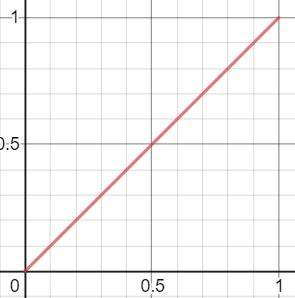``````Mostly just a test function for rebase/debase. Will always return the same number as the first argument.
``````

### PARABOLIC``````The first form of "accelerative" interpolation. Due to the nature of parabolas, the frequency cannot be controlled and will always consistently rise from min to max. Options include the power, which is 2 by default. Powers equal to 1 will make the interpolation linear, powers less than one will provide "negative acceleration" (never decreases, increases at an ever-lower rate), while powers greater than one will provide "positive acceleration".

f(i, min, max, {power: 2})
``````

### SINE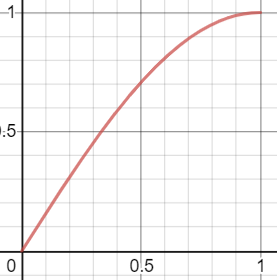``````The second form of "accelerative" interpolation. The frequency (times that the maximum value can be reached) can be controlled, which is also the rate of change. By default, it is 0.5. A frequency of 1 would, for example, change a number that is the mean of the minimum and maximum value to the maximum value itself, while numbers closer to the minimum and maximum values are the least, meaning that it would interpolate "upwards", then "downwards" half-way through. This can be desirable in certain situations.

f(i, min, max, {frequency: 0.5})
``````

### REFLECTIVE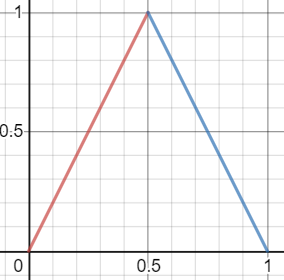``````A form of interpolation that is linear, but the rate of change "bounces" a specified number of times. Options include the frequency (bounce count), which is 2 by default.

f(i, min, max, {frequency: 2})
``````

### SAWTOOTH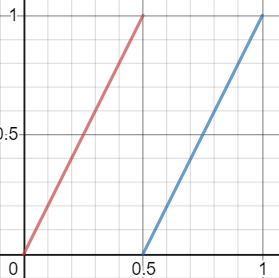``````A form of interpolation that is linear, but transforms directly from max to min a specified number of times. Options include the frequency (wrap count), which is 2 by default.

f(i, min, max, {frequency: 2})
``````

### SQUARE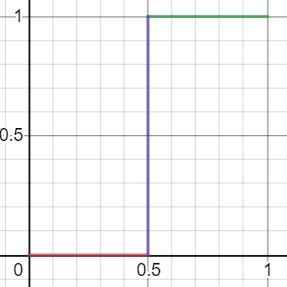``````A form of interpolation that will start at either 0 or 1 (polarity), and switch between 0 and 1 a certain amount of times (frequency) in an equally-spaced way. A frequency of two will make any value before 0.5 equal to (0 or 1), and any value that is or is after 0.5 equal to (1 or 0).

f(i, min, max, {frequency: 2, polarity: 0})
``````

### HERMITE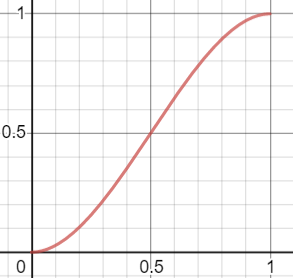``````Basic "hermite" interpolation. Good choice for preserving linearity while also introducing curvature.

f(i, min, max)
``````

## Keywords

### Install

`npm i niims`

8

1.0.3

ISC

8.58 kB

11